# Two-body problem

A problem dealing with the motion of two material points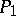and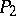with masses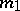and, respectively, moving in three-dimensional Euclidean space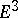when acted upon by the mutual Newton attracting forces. The problem is a special case of the-body problem, which may be described by a system of ordinary differential equations of order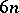, and has 10 independent integrals: 6 of motion of the centre of inertia, 3 of law of areas (equivalently, conservation of angular momentum) and 1 of energy conservation . The two-body problem also has three Laplace integrals (one of which is independent of the preceding ones) and is completely integrable .

The integration of the two-body problem is more conveniently effected in a special system of coordinates, in which these integrals are employed. If the origin of the Cartesian coordinates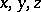is placed at the centre of massand the axisis directed along the relative angular momentum vector, then the motion of the relative position vector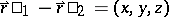takes place in the plane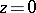and satisfies the system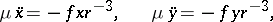(1)

where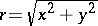,is the reduced mass andis the gravitational constant. The system (1) has four integrals: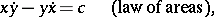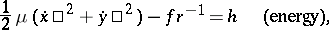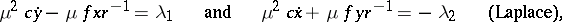which are interconnected by the relation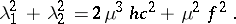Here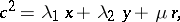(2)

i.e. the orbits of the relative position vector are conical sections with parameter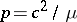, major semi-axis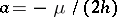, eccentricity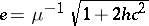, longitude of pericentre(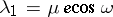,), and with focus at the coordinate origin. The location of the relative positive vector on the orbit is determined by the true anomaly, counted from the direction towards the pericentre; (2) then implies that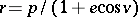. If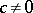, three types of orbits are possible:

I) If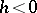, they are ellipses.

II) If, they are hyperbolas.

III) If, they are parabolas.

If, the motion is rectilinear. The two-body problem describes an unperturbed Kepler motion of a planet with respect to the Sun or of a satellite with respect to a planet, etc.